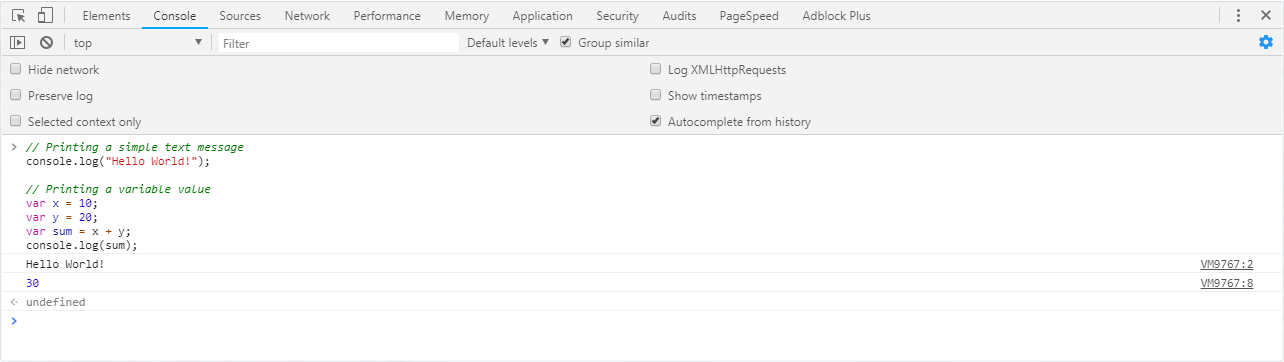# JavaScript 生成输出

## 将输出写入浏览器控制台

// Printing a simple text message
console.log("Hello World!"); // Prints: Hello World!

// Printing a variable value
var x = 10;
var y = 20;
var sum = x + y;
console.log(sum); // Prints: 30## 在警报对话框中显示输出

// Displaying a simple text message
alert("Hello World!"); // Outputs: Hello World!

// Displaying a variable value
var x = 10;
var y = 20;
var sum = x + y;


## 将输出写入浏览器窗口

document.write() 只有在解析该文档时，才可以使用该方法将内容写入当前文档。这是一个例子：

// Printing a simple text message
document.write("Hello World!"); // Prints: Hello World!

// Printing a variable value
var x = 10;
var y = 20;
var sum = x + y;
document.write(sum); // Prints: 30


<h1>This is a heading</h1>
<p>This is a paragraph of text.</p>

<button type="button" onclick="document.write('Hello World!')">Click Me</button>


## 在 HTML 元素中插入输出

<p id="greet"></p>
<p id="result"></p>

<script>
// Writing text string inside an element
document.getElementById("greet").innerHTML = "Hello World!";

// Writing a variable value inside an element
var x = 10;
var y = 20;
var sum = x + y;
document.getElementById("result").innerHTML = sum;
</script>